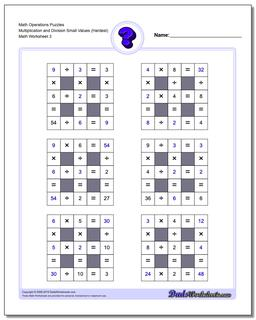# Math Worksheets: Number Grid Puzzles: Number Grid Puzzles: Math Operations Puzzles Multiplication and Division Small Values (Hardest) (Third Worksheet)## Math Operations Puzzles Multiplication and Division Small Values (Hardest) (Third Worksheet)

PropertyValue
DescriptionMath Operations Puzzles Multiplication and Division Small Values (Hardest): Math puzzle worksheets that require students to fill in missing numbers with values up Small Numbers. (Third Worksheet)
Resource TypeWorksheet